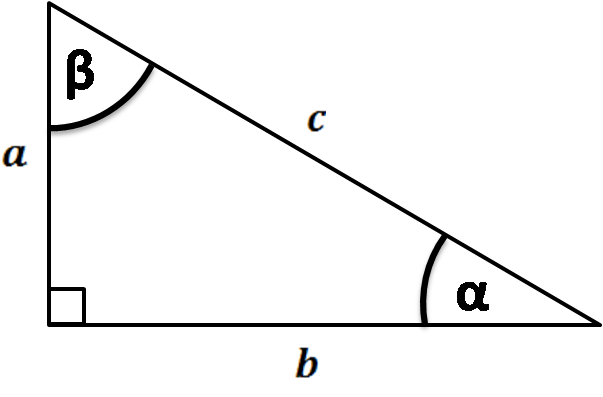# Cosine Triangle Calculator

Created by Arturo Barrantes
Reviewed by Dominika Miszewska, MD, PhD candidate
Last updated: Jun 09, 2022

The powerful cosine triangle calculator (also cos calc) is a tool that determines the cosine of an angle that can be expressed in degrees, radians, or pi radians—wondering how you will solve that trigonometry homework? Don't worry. In this article, we will explain what cosine is, how to calculate it, and will show several examples.

## What is the cosine in Trigonometry?

The cosine of an angle is a trigonometric function that relates both triangle sides that build the angle. It's better to explain it with an image:As you can see, $\alpha$ is the angle between the $b$ and the $c$ side of the triangle. Then, the cosine of alpha is the division between $b$ and $c$.

Note that $c$ is the hypotenuse of the triangle. So the cosine definition can be expressed as follows:

In a right triangle, the cosine is the trigonometric function that divides one side of the angle and the hypotenuse. Such side and the hypotenuse are the ones that build the angle we are referring to.

## How to calculate the cosine of an angle?

To calculate the cosine is pretty straightforward. You can use our cosine calculator or do the following:

1. Pick the hypotenuse value and the other side that builds the angle. In the above picture, we have represented them as $b$ and the $c$.
2. Divide $b$ by $c$. The result is the cosine of the angle. Note value can never go over 1.

## Cosine triangle example - What is the cosine of 30 degrees?

It is 0.866. Also, it is the square root of three divided by 2. How? Try Omnicalculator's tool cosine triangle calculator or do the following:

1. Recognize you have a 30-60 right triangle. This one is special because all the sides are in constant relationship. The hypotenuse is equivalent to 2; meanwhile, the other sides are equivalent to 1 and $\sqrt{3}$
2. The 30 degrees angle is built by the $\sqrt{3}$ side and the hypotenuse. Thus, we divide both sides and get the cosine: 0.866.

## Other useful tools like the cosine triangle calculator

Since you already know how to use this cosine calculator, you can take a look at other calculators:

## FAQ

### How do you get the cosine of an angle?

Try Omnicalculator's tool cosine triangle calculator or do the following:

1. Pick the hypotenuse and the other side that builds the angle. Let's call it c and b, respectively.
2. Divide the side by the hypotenuse, which means b by c.
3. The result is the cosine of the angle. Verify you do not get a result over 1.

### What is the cosine of 45 degrees?

0.707. We get it by dividing the side of a 45 degrees right triangle (equivalent to 1) and its hypotenuse (equivalent to the square root of 2).

Arturo Barrantes
Trigonometric function: Cosine
Angle
deg
People also viewed…

### Central angle

The central angle calculator finds the angle at the centre of a circle whose legs (radii) extend towards an arc along the circumference. Using two of the three parameters (central angle, radius, or arc length) find the third!

### Christmas tree

Welcome to the Christmas tree calculator, where you will find how to decorate your Christmas tree in the best way. Take a look at the perfect Christmas tree formula prepared by math professors and improved by physicists. Plan in advance how many lights and decorations you'll need!

### Reference angle

The reference angle calculator finds the corresponding angle in the first quadrant.